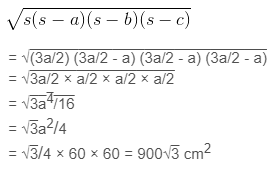Guru

# A traffic signal board, indicating ‘SCHOOL AHEAD’, is an equilateral triangle with side ‘a’. Find the area of the signal board, using Heron’s formula. If its perimeter is 180 cm, what will be the area of the signal board?

• 0

This question is from class 9 maths 12th chapter. the chapter name is herons formula. It is very important question. I want to get the solutions of this question because its very important. In this question they have asked .A traffic signal board, indicating ‘SCHOOL AHEAD’, is an equilateral triangle with side ‘a’. Find the area of the signal board, using Heron’s formula. If its perimeter is 180 cm, what will be the area of the signal board?

Share

1. Given,

Side of the signal board = a

Perimeter of the signal board = 3a = 180 cm

∴ a = 60 cm

Semi perimeter of the signal board (s) = 3a/2

By using Heron’s formula,

Area of the triangular signal board will be =• 0
2. i. 135 and 225

As you can see, from the question 225 is greater than 135. Therefore, by Euclid’s division algorithm, we have,

225 = 135 × 1 + 90

Now, remainder 90 ≠ 0, thus again using division lemma for 90, we get,

135 = 90 × 1 + 45

Again, 45 ≠ 0, repeating the above step for 45, we get,

90 = 45 × 2 + 0

The remainder is now zero, so our method stops here. Since, in the last step, the divisor is 45, therefore, HCF (225,135) = HCF (135, 90) = HCF (90, 45) = 45.

Hence, the HCF of 225 and 135 is 45.

ii. 196 and 38220

In this given question, 38220>196, therefore the by applying Euclid’s division algorithm and taking 38220 as divisor, we get,

38220 = 196 × 195 + 0

We have already got the remainder as 0 here. Therefore, HCF(196, 38220) = 196.

Hence, the HCF of 196 and 38220 is 196.

iii. 867 and 255

As we know, 867 is greater than 255. Let us apply now Euclid’s division algorithm on 867, to get,

867 = 255 × 3 + 102

Remainder 102 ≠ 0, therefore taking 255 as divisor and applying the division lemma method, we get,

255 = 102 × 2 + 51

Again, 51 ≠ 0. Now 102 is the new divisor, so repeating the same step we get,

102 = 51 × 2 + 0

The remainder is now zero, so our procedure stops here. Since, in the last step, the divisor is 51, therefore, HCF (867,255) = HCF(255,102) = HCF(102,51) = 51.

Hence, the HCF of 867 and 255 is 51.

• 0